# Brains as Analog Computers

## Brains might compute, but not digitally.

Here’s a good question: Is the brain a computer? One thing that makes this a good question is that it invites many further questions. Some people have taken the idea to be metaphorical: the brain is a computer in the same way that Juliet is the sun. That is just to say that it can be an illustrative way to think or talk about something, but not to be taken literally.

However, many do take it literally. My favorite example comes from the first sentence of Christoph Koch’s book, Biophysics of Computation: “Brains compute!’’ So what does it mean if we take this idea literally, too? What could it mean?

In what follows, I’ll briefly look at some previous ideas about how brains might compute, then explore the role of analog computation for making sense of neural computation.

## Algorithms

One idea, explored in a previous blog post in this very forum, is that the brain is a computer because what it does can be described in terms of algorithms. Unfortunately, this view has some problems. One big one is that lots of things (maybe even everything) can be described algorithmically. If that’s true, then of course the brain is a computer, because everything is. But ideas that are trivially true aren’t very interesting.

Maybe it’s not enough that something is merely describable by an algorithm for it to be a computer. Perhaps we can say that the brain computes because it follows, or runs, algorithms. Certainly not everything does that, even if everything is describable by an algorithm. Still, there is a problem with this idea. There’s a subtle, but important, distinction between being algorithmically describable and actually following an algorithm.

Here’s an example. Suppose I ask a child to write down a pattern of numbers, using the following rule. Start with 1, then add 3 to it and write that down, then add 5 to what you just wrote down, then add 7 to what you just wrote down, and so on. The child writes down 1, then 4, then 9, then 16, etc.
Clearly, the child is following an algorithm — the “add 3, then 5, then 7, etc.” rule.

But we can describe what the child is doing in terms of another algorithm: the child is producing the squares of consecutive integers. There are two different algorithms that produce the same pattern (in fact, there are infinitely many such algorithms). In this example, we know which algorithm the child is following out of the many algorithms that describe the behavior. But in other cases, we might not know at all.

In fact, it might not even make sense to think that the behavior of an organism is produced by following an algorithm at all, even if it is describable by some algorithm. For example, do single-celled organisms that move through increasing chemical gradients toward food really follow an algorithm? We know that computers can run algorithms depending on which program is running: these algorithms are stored and represented within the system. But for single-celled organisms, the “algorithm” is probably not represented anywhere: it’s just what the system does. But maybe it’s not a requirement that systems explicitly store and represent the algorithms they follow; however, that implies that objects falling due to gravity also follow algorithms! This does not seem like a happy result. These are all difficult and interesting questions, but for now, I will set them aside.

A different problem with the idea of following algorithms is that what we usually mean by “algorithm” is entirely discrete. An algorithm consists of a finite series of discrete instructions, each of which takes some discrete amount of time. Turing’s work on the mathematical analysis of algorithms — and thus computation — assumes discrete time-steps and discrete variables (although, to be sure, “time’’ has to be understood abstractly as simply a succession of events, one after another, without particular units, such as milliseconds). Modern digital computers make the same assumptions. But we know that many elements of the brain are not discrete: there are plenty of continuous quantities that seem to have an impact on what neurons do.

So here’s another problem. How we normally understand algorithms and computation is discrete through-and-through, but we know brains sometimes use continuous variables and processes. Even though we can simulate continuous quantities digitally, that does not mean that continuous processes just are discrete.

There are other problems, but rather than going through them all, I think it’s better to look at a different way forward. But to get there, we have to take a moment to think carefully about what analog really means, and what discrete and digital mean — as well as how they can come apart. The upshot is that we can re-discover a way to think about computation that can be applied to computation in the brain.

## Analog Representation

Thinking of the brain as an analog computer makes a lot of sense, but first we have to be clear about what exactly that means. Some have entertained this idea, but under the mistaken idea that “analog” is just synonymous with “continuous.” One thought along these lines is that, because continuous quantities can be simulated digitally, analog computation is not worth taking seriously. However, there is much more to analog computation, and for those of us who want to understand how (or even if) brains compute, we should try to understand different types of computation.

First, we need to get a handle on analog representation.

When most people think of what “analog’’ means, they think it just means continuous. In fact, the terms “analog’’ and “continuous’’ are often used interchangeably (although sometimes people also use “analog’’ to mean not-digital, or not on a computer, which is too bad). However, a bit of reflection, plus a closer look at how analog computers actually operate, shows that this is not right. Instead, here’s the key idea:

Analog representation is about covariation, not continuity.

Let’s start with some examples of simple analog devices. A mercury thermometer is a good one (although mercury has largely been replaced by alcohol). What makes this kind of thermometer analog, rather than digital? The way it works is simple: the thermometer represents temperature, and as the temperature increases, so does the level of liquid in the thermometer.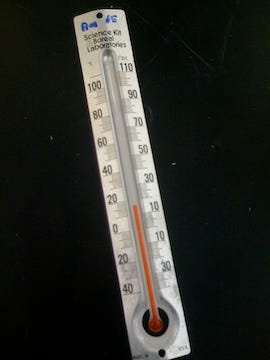An analog thermometer.

Another example is the second hand of an analog clock. The way it works is also simple: the hand represents time, and as time increases, so does the angle of the second hand.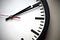An analog clock: as time increases, so do the angles of the hands.

In both of these examples, the device represents something: temperature for the thermometer, and time for the watch. Also, in both of these examples, that representation is analog. Why? Simply put, because there is an analogy between the representation and what it represents. Specifically, as the thing that’s being represented increases, the physical property that’s doing the representing also increases. And by increase, I mean a literal increase: an increase in the height of the liquid in the thermometer, and an increase in the angle of the second hand (with respect to 12, or straight up).

But angles and heights are continuous, right? I just said that continuity is not what being analog is all about. But think about that analog clock again. Some electric clocks have second hands that sweep continuously, but many analog clocks (such as wristwatches) tick: the second hand moves in discrete steps. Does ticking (i.e. moving in discrete steps) mean an analog watch isn’t really analog anymore? Of course not! An analog representation can be continuous or discrete, as long as the right kind of physical covariation is in place. When you start looking for them, you can see many more examples, too. Hourglasses, for example, are analog representations of how much time has passed, whether they contain a liquid, really small particles that you just take to be continuous, or big, discrete things like marbles.

Now this is all just a matter of thinking about the concept of “analog,” and looking at some examples of analog representation. But it also turns out that this is how to understand analog computers.

## Analog (vs. Digital) Computation

If you aren’t familiar with analog computation, you’re not alone. It was once the dominant computing paradigm, but digital computers have almost completely replaced analog computers. With advances in engineering, digital computers eventually became faster, more flexible, and cheaper than their analog counterparts. Nevertheless, they’re fascinating, and not just as a historical curiosity. They also exemplify a completely different kind of computation that — although not practical from an engineering perspective — shows another way that brains might compute. So let’s take a brief look at how they work.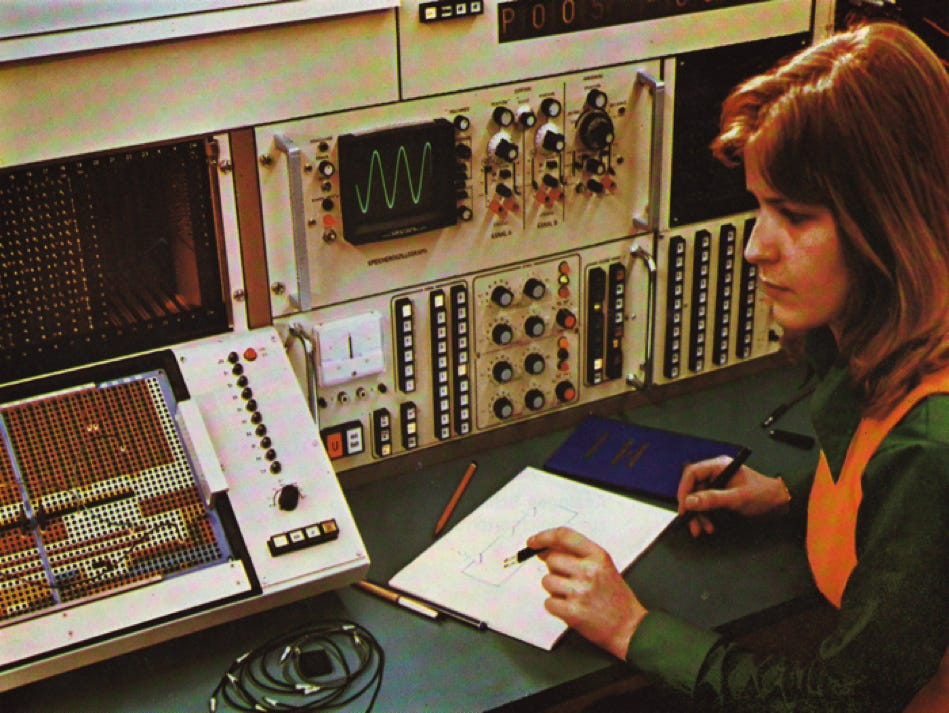Engineer operating the Telefunken 770 RA analog computer.

The key idea of analog computers is that they represent variables by the actual voltage level of a circuit element. So if you have a variable with the value 72.3, the circuit element representing that variable would be at 72.3 volts. This is completely different than how such a value would be stored in a digital computer: in that case, 72.3 would be represented by a series of 1s and 0s in some register (or, according to the IEEE 754 standard for floating-point numbers, 01000010100100001001100110011010).

To add two variables in an analog computer, you use a circuit that literally adds the voltages: a circuit element would take two inputs, one that has x volts, one that has y volts, and produce an output that has (x+y) volts. But in a digital computer, to add two variables you use circuitry that adds the two numbers digit-by-digit, much the way that we all learned how to add numbers in elementary school. The least significant digits are added first, then the next most significant (plus a carry digit from the previous addition, if needed), and so on, until we reach the end of the digits.

A lot of the variables in analog computers are continuous, but there are exceptions, and those exceptions are important. Often, when using an analog computer, it helps to know how to program it using a mathematical characterization of whatever you’re interested in. But there are times when you might not know how to characterize something mathematically: you just know what it looks like. So instead of using a continuous function like a sine wave or polynomial, analog computers could approximate complex curves with a series of straight line segments.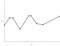A continuous function (gray) approximated by a series of line segments (black).

Other times, they would use step functions, with gaps between one value and another, where the voltage would literally switch between the values. Did the existence of these discontinuities mean that these computers weren’t really analog? Not at all: just like analog clocks that tick, analog computers with “steps” are still analog. And again, the reason is that there is analogy between what they represent and how they represent it.

The point is worth belaboring a bit, especially in contrast with digital representation. Somewhat paradoxically, digital representation is both much more complicated but also much more familiar.

Let’s take two digital representations of two different numbers. To keep things simple, we’ll use base-10, which we’re all familiar with, instead of the binary, or base-2 representation used in digital computers. The point is the same in either case. Compare how we represent the number three hundred forty seven and the number seven hundred twelve. Digitally, we represent the first number as 347, and the second as 712. What do those strings of numerals mean? Again, we are so familiar with this that we rarely stop to think about it, but we interpret them as follows:

347
= (3 × 10²)+(4 ×10¹)+(7 × 10⁰)
= (3 × 100)+(4 ×10)+(7 × 1)
= 300 + 40 + 7

712
= (7 × 10²)+(1 ×10¹)+(2 ×10⁰)
= (7 × 100)+(1 ×10)+(2 × 1)
= 700 + 10 + 2

An important thing to note is that when we compare the two representations, neither one is larger than the other. Of course, seven hundred twelve is a larger number than three hundred forty seven. But the three character string “712” is not itself larger than the three character string “347” (as long as we hold the font fixed!).

Things are different when it comes to analog representations. If we represent these two numbers in an analog computer, for example, one voltage (712 volts) is literally larger than the other (347 volts). Or in the case of the analog thermometer, the height of the liquid representing 80 degrees is literally taller than the height representing 60 degrees.

Again, all of this still holds whether or not voltages and heights can only come in discrete pieces; analog representation just doesn’t have anything to do with continuity.

Before continuing, let me mention another point about what digital does not mean. Some people take “digital” to be synonymous with “discrete,” but the two are different. Digital representations are, well, representations of digits, just like the example we just went through. “Discrete,” however, is much more general, and just means that the thing in question has separate parts. For many purposes, it may not be important that we’re careful about the distinction, but when we’re talking about computation, either in the brain or elsewhere, it is very important. Why? Simply because digital computers use the fact that numbers are represented digitally in order to function as they do. They are not called digital computers simply because they use discrete elements, or operate in discrete steps, but because they represent numbers (including variables, memory addresses, instructions, and so on) in base-2, digital format.

Now, different kinds of tasks are better served by different kinds of computers using different kinds of representations. As it happened, digital computers got fast enough and cheap enough that they were preferred over their analog counterparts, although that wasn’t always true. But let’s look at just one simplified example to show the difference between a digital and an analog computation.

Suppose I give you one thousand numbers that are represented digitally. More specifically, suppose I give you a thousand index cards, each one of which has a single number written on it. Your task is to find the largest number in that stack of a thousand. The fastest way to do this is also the simplest: you take the first card, call it the largest-so-far, then compare it with the next card. If that card is larger, you have a new largest-so-far; if not, you don’t. You keep comparing, and after 1,000 steps, you will have found the largest card in the pile. In general, how many steps does it take? It takes as many steps as cards you have. In computational complexity theory, we would say that this task has linear time complexity: start with 2,000 cards, it will take you twice as long; 3,000 cards, three times as long.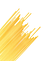Spaghetti noodles. © Can Stock Photo / AlfaStudio

Now, suppose that instead of giving you a thousand numbers represented digitally, I gave you a thousand analog representations of numbers. In particular, suppose that I give you a bundle of one thousand spaghetti noodles, where the length of each noodle (in, say, millimeters) is the number being represented. Your task (again) is to find the largest number in those thousand. The fastest way to do this (again) is also the simplest: you take the bundle of noodles, tap one end on a flat surface, like a table, and place your hand down so that it hits the tallest one. After a single step, you will have found the largest noodle in the bundle, which represents the largest number of the thousand. In general, this only takes one step, which is constant time complexity (much better than linear!). No matter how many numbers (or, rather, representations of numbers) you start with, it’s always just one step.

This example illustrates one way that analog representation can be more efficient, but it also illustrates one of its limitations. Say we had many numbers that were very close in value to one another; it may be difficult to pick out the tallest one if they only differ by fractions of a millimeter. Represented digitally, however, we can easily tell whether two numbers are different. When it comes to contemporary digital computers, where individual steps can be taken at the rate of billions per second, this increased precision outweighs the larger number of steps needed (this, among others reasons, is why analog computers have fallen out of favor for general use).

## Analog Computation in General…

At this point, I hope to have made clear what analog representation is, and given at least a flavor of how analog computation works. Next, I need to say more about analog computation in general. Luckily for us though, understanding analog representation is the hard part. All we need to add to the story to get analog computation is a mechanism that manipulates analog representations. But not just any old mechanism will do, nor will just any old manipulation. We need to be more specific.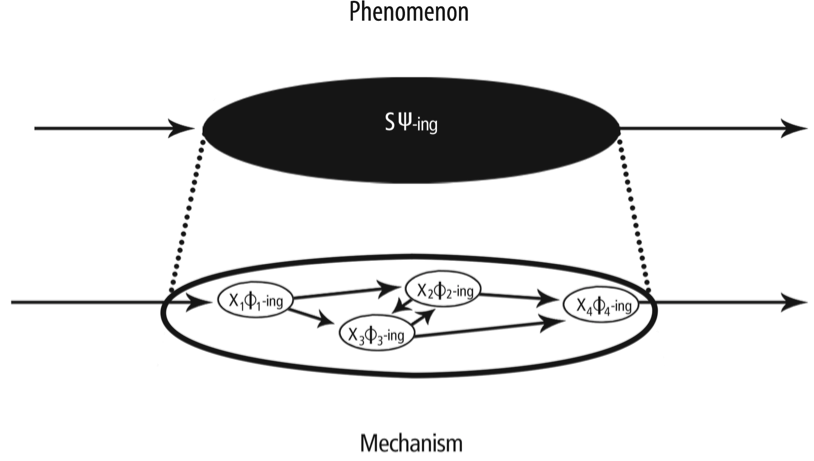Schematic diagram of a mechanism: organized entities and their activities (bottom) are responsible for a phenomenon of interest (top).

Philosophers of science have developed an account of mechanisms that makes precise what scientists, especially neuroscientists, implicitly mean when they talk about mechanisms (a book-length account is given in Carl Craver’s book Explaining the Brain). We don’t need to get into the details, but the general idea is straightforward: a mechanism is a set of entities and activities, organized in a particular way, that give rise to a phenomenon of interest. For much of neuroscience, what it means to explain some phenomenon is to discover and describe the mechanism responsible for that phenomenon. This is in contrast to, say, physics, where explanation involves describing a universal law of nature.

So if we have a mechanism that manipulates analog representations, do we have an analog computer? Not quite. The manipulation has to be of the right kind. For example, I could build a device that rotates an analog thermometer (like the thermometer mentioned above). That’s certainly a kind of manipulation, and the device that does the rotating may well be a mechanism. But it’s not the right kind of manipulation. So what is the right kind?

In short, the mechanism has to manipulate the part of the analog representation that is doing the representing. So when we want to represent a temperature, we have to manipulate the height of the liquid in the thermometer, not its angle. This, by the way, is precisely how thermostats work: one part of the device represents the actual temperature, another part of the device represents the desired temperature. And for analog thermostats, this is done with analog representations.

Before we move on to see what this has to do with brains, let me point out that a nice thing about the story I just told is that it generalizes pretty well to digital computers, too. Just replace “analog” in what I said above with “digital:” a digital computer is a mechanism that manipulates digital representations, and it also has to manipulate them in the right way. Heating up the circuitry on your laptop is definitely a way to manipulate the digital representations inside, but not in a way that constitutes computation.

## …And in the Brain

Okay, now that we know what analog computation is, what does this have to do with brains? Quite a lot!

First, a general point. Couching computation in terms of representation helps us distinguish what’s computational about the brain, and what isn’t. Brains, like all organs, do all kinds of things that aren’t directly relevant to their primary function, but simply help keep them alive. So for example, we once thought that glial cells only held neurons together and didn’t contribute anything interesting to neural signaling (hence their name, derived from the Greek word for glue). We now know that at least one type of glial cell, the astrocytes, do contribute to signaling between neurons; another type, the ependymal cells, do not. That means that astrocytes — but not ependymal cells — contribute to computation in the brain. Neural signals are representations (or parts of representations), and the manipulation of those representations (by the right kind of mechanism) is computation.

But let’s talk more specifically about what the analog part of this story about computation has to do with brains. There is a whole lot of neural activity that counts as analog representation; you just have to remember that analog representation is about covariation (as discussed above), and not necessarily about continuity. So let’s look at some examples.

First, consider rate coding, one of the most well-studied ideas of neural representation, and also one of the earliest. The basic idea of rate coding is simply that as a stimulus intensity increases (or decreases), the firing rate of the relevant neuron increases (or decreases). In other words, the representation (firing rate) increases with the thing being represented (the stimulus). That is about as straightforward an example of an analog representation as one could want. Whether it then counts as analog computation depends on whether the system in question manipulates that representation. For example, in their seminal 1926 work, Adrian and Zotterman found that as they increased the weight attached to muscle tissue, the sensory neurons of that muscle tissue increased their firing rate. The firing of those neurons serve as input to downstream neurons, and we have an analog computation.

Now, rate coding has its limitations, but we can apply the model of analog computation to other neural coding schemes, too. For example, consider timing codes. Some timing codes in the auditory system, for example, work by comparing the relative time that different neural signals arrive at the same place. This allows the organism to locate where a sound came from. The larger the distance between the arrival of two signals, the larger the angle of the sound’s location from the center. Once again, an analog representation, used by the system, resulting in an analog computation.

A more complicated example is how grid cells work. These are groups of neurons that create a two-dimensional map of a two-dimensional environment. So, for example, as the organism moves right, the activity of the grid cells “moves” right; as the organism moves left, the activity “moves” left. (More precisely, neurons representing locations to the left of the current position will fire as the organism moves left, vice versa for the right.)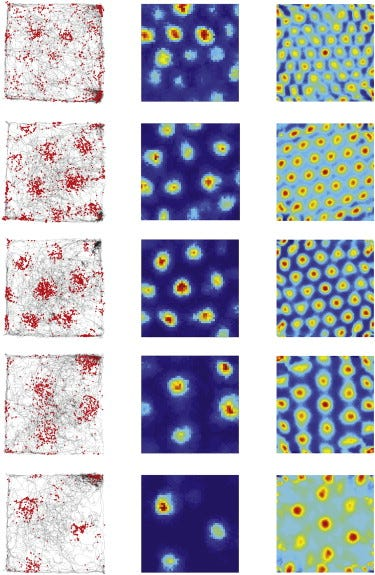Grid cells firing in response to an organism’s movement.

This is an example of a two-dimensional analog representation, rather than the one-dimensional examples from above. Instead of changing just up or down, increasing or decreasing, we have change along two spatial dimensions. And the change in what’s represented (the environment) results in a corresponding change in the representation (the grid cells).

Another, higher-level, example is mental rotation in humans, which relies on the manipulation of analog representation (which, if you buy the view I propose here, just is analog computation). Here is the task used in the relevant studies, originally devised by Shepard and Metzler in 1971. A participant is shown two pictures of 3-D objects, and asked to push one button (“same”) if the one on the right is a rotated version of the one on the left, and a different button (“different”) if the one on the right is a different object. An example is in the figure below: the top two figures are “same,” but the bottom two are “different.”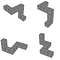Mental rotation stimuli. Top two objects are “same,” while the bottom two are “different.”

Interestingly, when you record the time it takes for people to make a response (we only care about the “same” ones), you find that the more the objects are rotated, the longer it takes people to make that response. It’s as if people are mentally “rotating” the object in their head, and checking to see if the objects match. So, the more the objects are rotated, the more mental rotation they have to do, which translates into a longer response time.

This finding has been replicated in numerous studies; in recent decades, cognitive neuroscientists have produced fMRI data from people performing the task while having their brain scanned. In a meta-analysis from 2008, Jeff Zacks found that dozens of these studies support the view that mental rotation depends on analog representations, supporting the original hypothesis proposed by Shepard and Metzler. Why should we think this?

One important point is that there are much more efficient ways to rotate the representation of an object. Using a typical digital representation, such as what is used in computer graphics systems, involves linear algebra. Without going into the details, the idea is that we can — in one step — multiply the 3-D coordinates of an object by a matrix, resulting in the object being rotated. Importantly, the amount of time it takes to rotate an object by two degrees is the same amount of time it takes to rotate an object by 180 degrees. However, that is simply not the result we find when humans perform this task. Instead, longer rotations take more time. That suggests that we are not rotating the object in a single step, but manipulating an analog representation that covaries with what it represents.

An analogy helps. Think about adding a couple of two-digit numbers the way you learned in elementary school. To keep things simple, we’ll use numbers that don’t require any carry digits. So if we want to add 11 to 12, we put one on top of the other, and add the digits. Same thing if we want to add 66 and 33.

In each case, it takes the same number of steps, even though in the left problem, we’re starting and ending with much smaller numbers. This is just a fact about doing the addition digitally: even though the numbers are bigger, we’re just manipulating digits, and we’ve got the same number of digits in each case.

But let’s say we had to do the addition in a way that you learned when you were even younger, using (although you didn’t know it at the time) analog representations. Suppose we had a big bag of marbles, and we did the problem on the left by taking out 11 marbles, one at a time, then adding 12 marbles to those, one at a time, and then counting how many marbles we end up with. That would obviously take much less time than doing the problem on the right the same way. Now granted, this is not an efficient way of doing addition! But it does illustrate how analog — but not digital — representations take longer time to do some computations.

At this point, some might think that this is all well and good, but at the very lowest levels, neural spikes are like the bits of digital computers; so maybe this analog stuff doesn’t have much to do with the hardware of the brain. Neural spikes are either on or off, just like the 1s and 0s of digital computers. John von Neumann, one of the founders of the digital computer and a prolific polymath, put the view like this in his 1957 lecture: “The nervous pulses can clearly be viewed as (two-valued) markers: the absence of a pulse then represents one value (say, the binary digit 0), and the presence of one represents the other (say, the binary digit 1). This is clearly the description of the functioning of an organ in a digital machine. It therefore justifies the original assertion, that the nervous system has a prima facie digital character.” So maybe there are some analog things happening at higher levels, but at its root, neural spikes are discrete and digital.

However, some new evidence suggests that this might not be the whole story. An intriguing set of examples from scientists including Bialowas, Rama, Rowan, and several others shows that there may be more to action potentials than previously thought. So first, let’s review a bit about action potentials, then see what these new results suggest.

The traditional view of the action potential is that it is a lot like the binary pulse of a digital computer. If we look close at the 1s and 0s of a digital computer, we’ll see that they are actually continuously-changing voltages. However, that continuous change stays around either (for example) zero volts or five volts, and the minor fluctuations above and below those two levels don’t matter for digital systems. That’s because we have designed them that way: even though there is continuous fluctuation, we can treat those voltages as if they are really at two discrete levels, which we call 0 and 1. The slight difference in the waveform from one bit to another doesn’t matter: all that matters is that there is some voltage that’s pretty close to 5 volts or not.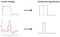The digital computer and the neuron. Top: actual transistor voltage is “translated” as a 1. Bottom: actual neuron voltage is “translated” as a 1.

This is how neuroscientists have traditionally viewed the action potential, too. If we compare two different action potentials, there might be a slight difference in the waveform, but that doesn’t matter for the system. All that matters is whether there’s an action potential or not. Now, to be sure, there are exceptions: some neurons don’t generate spikes at all, but have a signal that varies continuously — neurons connected by gap junctions are an important example. And for other neurons, it’s not really the single spike that matters, but their rate of firing, as mentioned above. But these new findings are different altogether.

Instead of having no significance, the scientists mentioned above have shown that the precise shape of the neural spike does have consequences. What does that mean? Basically, if a neural spike is a bit taller (it has a higher voltage), then it has a measurable effect on what happens to the neurons it’s connected to. Or, if the spike is a bit wider (it takes a little bit longer), then it also has a measurable effect on downstream neurons. These effects are small, but they are measurable, and completely different than what we find in digital computers.

So do these count as analog representations? Well, we don’t know yet. They are candidates, because we have something (a neural spike) that varies in the right way. But we do not yet know if these are representations at all. As mentioned before, neurons can do a lot of things, not all of which contribute to their representational capacities. If it turns out that the height (or width) of the neural spike increases as some other variable increases, it may well be a representation. We will have to see. For now though, it is an interesting candidate.

Finally, let me mention one aspect of analog computation that really has no counterpart in digital computation, which is also, admittedly, the most speculative on my part. Imagine you have a small computer program, or perhaps even a spreadsheet, where you have some variable called, say, “GrandTotal.” It’s easy enough to program a computer (or create a spreadsheet) that adds a whole bunch of numbers together, and stores that result in GrandTotal. And somewhere, deep in the electronic bowels of your computer’s processor, there are some circuits called registers, and there’s a single register that physically stores the value of GrantTotal. Your computer is doing a lot of other things, so there are a lot of other values stored in nearby registers, too. Suppose, in fact, that, just for fun, you wanted to add the values of the eight nearest neighbors — the other registers nearest to GrandTotal — and store those in GrandTotal, too. How can you do this?

Unfortunately, you can’t. The way digital machines are designed and built, their physical implementation is completely abstracted from their programming. There is no way to access variables that are literally, physically closest to the one you are working with. Of course, if you are very familiar with a particular computer, you might be able to discover which of those registers are closest. But then they will be completely different in another machine. There is simply no way to put this kind of capability into the general programming of a digital computer.

Interestingly, however, neurons do things like this all the time. Some neural signals, such as neuromodulators, are often simply broadcast to whatever neurons happen to be nearby. This capability takes advantage of the fact that neurons are physical devices, located in space relative to one another. And although digital computation can’t provide this kind of capability, certain kinds of analog computation can. This is simply because analog computation embraces the physical nature of its representations, whereas digital computation abstracts away from it. Now to be sure, digital computation has many advantages: it is quite nice to be able to use the same program on a wide variety of different computers from different manufacturers, with different speeds, different amounts of memory, and so on. But there is more to computation than just the digital, which, if I’ve done my job, you’ll believe now, too.

Analog computers have fallen out of favor, and as a consequence, we don’t think about them when we think about computation. And while the advantages of digital computation are clear for practical purposes, analog computation turns out to be an excellent way to think of computation more generally. When we look closely at how digital computation really works, it has almost nothing in common with how brains work. If digital computation is the only concept of computation you have, you might think we should abandon the idea that brains literally compute. But that would be much too hasty: we just need a broader notion of computation, and it turns out that looking to analog computation helps us see how brains could be computers after all.

Want more? Follow us at The Spike

## The Spike

The science of the brain, from the scientists of the brain

Written by

## Corey J. Maley

Philosopher of computation and neuroscience. www.coreymaley.net

## The Spike

The science of the brain, from the scientists of the brain

Written by

## Corey J. Maley

Philosopher of computation and neuroscience. www.coreymaley.net## The Spike

The science of the brain, from the scientists of the brain

## More From Medium

Medium is an open platform where 170 million readers come to find insightful and dynamic thinking. Here, expert and undiscovered voices alike dive into the heart of any topic and bring new ideas to the surface. Learn more

Follow the writers, publications, and topics that matter to you, and you’ll see them on your homepage and in your inbox. Explore

If you have a story to tell, knowledge to share, or a perspective to offer — welcome home. It’s easy and free to post your thinking on any topic. Write on Medium